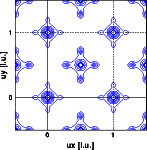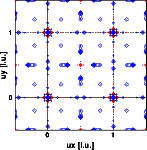Structure solution

Goto
Contents

The Patterson syntheses calculated in the last part were:Why is the Patterson density a periodic function in real space ?

• A Patterson shows the interatomic vectors of the structure. Since the structure itself is periodic, the distribution of interatomic vectors is periodic as well. You can deduce this straight from the formula:The complex exponential is identical for the argument mod 2 PI, or for hu mod 1. Thus, if an integer vector u is added, the result remains invariant. Note that this holds only if h is restricted to integer values.
Explain the differences between the X-ray and neutron Patterson.
Why are the neutron Patterson peaks at 1/2 1/2 0 and at 0.2 0 0 negative ?

• Each peak in the Patterson represents an interatomic vector. Its height is determined by the number of identical vectors and by the product of the scattering factors of the two atoms.
• The X-ray intensities are dominated by the metals. The oxygen contributes only a minor fraction to the intensities. Accordingly the X-ray Patterson is dominated by the metal-metal vectors.
• The neutron scattering lengths of the atoms do not increase with increasing atomic number. The scattering lengths of the elements in this example are:

 ``` Zirconium 0.69 Titanium -0.33 Oxygen 0.575 ```

• The peak at 1/2 1/2 0 represents the metal-metal vector. Since the scattering length of Titanium is negative, this peak is negative. Accordingly the peak at 0.2 0 0 represents the Titanium - Oxygen vector. Note that this distinction between the Ti-O and Zr-O could not be made as unambiguously from the X-ray Patterson.
Solve the structure, at least for the metal atoms.

• Using the Patterson peaks and the space group information the structure can easily be solved:

 ``` Zirconium 0.5 0.5 0.0 Titanium 0.0 0.0 0.0 Oxygen 0.2 0.0 0.0```

For the final structure determination go to the next section ..

© Th. Proffen and R.B. Neder, 2003